## Wednesday, January 30, 2019

### A variant of the transportation problem

In  a problem is described:

• We have $$n$$ groups of volunteers. Groups have different sizes (number of volunteers).
• There are $$m$$ volunteer sites with a certain demand for volunteers.
• Try to assign volunteers to sites such that we don't split up groups if not needed.
As is often the case, a verbal description of the problem gives rise to imprecision. Building a model helps us to surface those issues: it forces us to get the details right and have some consistency.

We can recognize a transportation model in the description of the problem. We have $$n$$ supply nodes (the volunteer groups) and $$m$$ demand nodes (the volunteer sites).

Transportation model
\begin{align} \min & \sum_{i,j} \color{DarkBlue} c_{i,j} \color{DarkRed} x_{i,j}\\ & \sum_j \color{DarkRed} x_{i,j} \le \color{DarkBlue}{\mathit{Supply}}_{i} &&\forall i \\ & \sum_i \color{DarkRed} x_{i,j} = \color{DarkBlue}{\mathit{Demand}}_{j} &&\forall j \\ & \color{DarkRed}x_{i,j} \ge 0 \end{align}

Here we assume total supply is equal or exceeds total demand. If total demand ls larger than total supply, we can use as constraints \begin{align}& \sum_j \color{DarkRed} x_{i,j} = \color{DarkBlue}{\mathit{Supply}}_{i} &&\forall i \\ & \sum_i \color{DarkRed} x_{i,j} \le \color{DarkBlue}{\mathit{Demand}}_{j} &&\forall j \end{align} In the special case where total demand is equal to total supply, we can use either formulation, or alternatively  \begin{align} & \sum_j \color{DarkRed} x_{i,j} = \color{DarkBlue}{\mathit{Supply}}_{i} &&\forall i \\ & \sum_i \color{DarkRed} x_{i,j} = \color{DarkBlue}{\mathit{Demand}}_{j} &&\forall j \end{align}

OK, we had to make one assumption. Besides that we have two more issues:

• The variables $$x_{i,j}$$ should be integer value: we cannot divide up a volunteer.
• The objective is not correct. We need somehow to model "split up as few groups as possible".  One interpretation could be: minimize the number of links $$i \rightarrow j$$ that we use. Putting it differently: find the sparsest solution for $$x_{i,j}$$.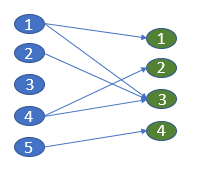Bipartite graph representation
A model that minimizes the number of used links is:

\begin{align} \min & \sum_{i,j} \color{DarkRed} y_{i,j}\\ & \sum_j \color{DarkRed} x_{i,j} \le \color{DarkBlue}{\mathit{Supply}}_{i} &&\forall i \\ & \sum_i \color{DarkRed} x_{i,j} = \color{DarkBlue}{\mathit{Demand}}_{j} &&\forall j \\ & \color{DarkRed}x_{i,j} \le \color{DarkBlue}x^{\color{DarkBlue}up}_{i,j} \cdot \color{DarkRed} y_{i,j} \\ & \color{DarkRed}x_{i,j} \in \{0,1,\dots, \color{DarkBlue}x^{\color{DarkBlue} {up}}_{i,j}\} \\ & \color{DarkRed}y_{i,j} \in \{ 0, 1 \} \\ & \color{DarkBlue} x^{\color{DarkBlue} {up}}_{i,j} = \min(\color{DarkBlue}{\mathit{Supply}}_{i},\color{DarkBlue}{\mathit{Demand}}_{j}) \end{align}

Let's test this with some random data:

----     18 PARAMETER size  volunteers in group

group1   47,    group2  212,    group3  140,    group4   79,    group5   76,    group6   60,    group7   91
group8  215,    group9   21,    group10 128,    group11 250,    group12 147

----     18 PARAMETER request  needed by site

site1 397,    site2 306,    site3  55,    site4 257,    site5  66

----     18 PARAMETER numvolunteer         =         1466  total volunteers
PARAMETER numrequest           =         1081  total requests


The solution can look like:

----     43 VARIABLE y.L  link used

site1       site2       site3       site4       site5

group2                        1
group3                                                1
group4                                                            1
group5                                    1
group8                        1
group10                                               1
group11           1
group12           1

----     43 VARIABLE x.L  flow

site1       site2       site3       site4       site5

group2                       91
group3                                              140
group4                                                           66
group5                                   55
group8                      215
group10                                             117
group11         250
group12         147

----     43 VARIABLE z.L                   =        8.000  objective


There are specialized algorithms for the transportation model. I often use LP solvers for this, as they provide a little bit more flexible and have very competitive performance. I don't think there is an algorithm for the "min number of links" problem. A MIP formulation seems appropriate.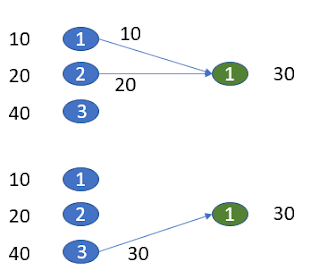Is minimizing number of links the best approach?

A disadvantage of my approach is that using a partial group has the same cost as using a complete group. The picture above illustrates the issue. Arguably one link related to incomplete use of a group may be not as good as two links but using up all supply. Of course if we have a balanced problem, i.e. total supply = total demand, there is no issue with this. In that case all groups will be used completely, so the second case (one link, but leaving leftover supply) will not happen. The question remains: what would be a better approach for unbalanced problems?

Well, one interesting fix is: add an extra dummy node that handles leftover demand or supply. In our case supple exceeds demand so we add an extra demand node. Its demand is previous total demand $-$ total supply. The picture becomes: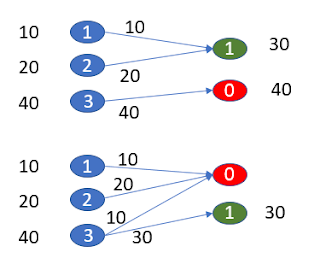First assignment is better: 3 active links vs 4

When we do this for our original data set we see:

----     47 VARIABLE y.L  link used

site1       site2       site3       site4       site5       dummy

group1                                                1
group2                        1           1
group3                                                1           1
group4                                                                        1
group5                                                1
group6                                                            1
group7                                                                        1
group8                                                                        1
group9                        1
group10                       1
group11           1
group12           1

----     47 VARIABLE x.L  flow

site1       site2       site3       site4       site5       dummy

group1                                               47
group2                      157          55
group3                                              134           6
group4                                                                       79
group5                                               76
group6                                                           60
group7                                                                       91
group8                                                                      215
group9                       21
group10                     128
group11         250
group12         147

----     47 VARIABLE z.L                   =       14.000  objective


This now solved a balanced problem, and more accurately counts if groups are split up. The optimization model is the same, just the data has been updated with a dummy demand node.

#### References

1. Algorithm for optimally matching certain sized volunteer groups with volunteer sites asking for specific numbers of volunteers?, https://stackoverflow.com/questions/54371450/algorithm-for-optimally-matching-certain-sized-volunteer-groups-with-volunteer-s

## Saturday, January 26, 2019

### Strange math

Some examples that caught my eye.

#### Strange constraints

In  we see a strange constraint:

A constraint like $0.8 x \le x \le 1.2 x$ effectively says: $$x\ge 0$$.

I think the poster means $0.8 x^0 \le x \le 1.2 x^0$ where $$x^0$$ are the original levels. In GAMS you can write this as:

e1.. x =l= 1.2*x.l;
e2.. x =g= 0.8*x.l;

or better

x.lo = 0.8*x.l;
x.up = 1.2*x.l;

New LP modelers often have problems considering the constraints as simultaneous equations. Many times I see they think about some ordering.

#### Valid math?

In  we see a constraint like: $p_{i,j}= \frac{u_{i,j} a_{i,j} y_j}{\sum_j u_{i,j} a_{i,j} y_j}\>\> \forall i \in I, j \in J$ Looks like some kind of normalization. I am not sure this is valid math: we have two different $$j$$'s. Probably we should apply some scoping rules when reading this. In GAMS we would see:

4  eq(i,j).. p(i,j) =e= (u(i,j)*a(i,j)*y(j))/sum(j,u(i,j)*a(i,j)*y(j));
****                                                 \$125
**** 125  Set is under control already

I agree with this error.

#### References

1. How to define variables, constrains to Pandas Dataframe when using CVXPY for optimization?, https://stackoverflow.com/questions/54336137/how-to-define-variables-constrains-to-pandas-dataframe-when-using-cvxpy-for-opt
2. How to use large data sets with non-linear optimization solvers in python?, https://stackoverflow.com/questions/54293666/how-to-use-large-data-sets-with-non-linear-optimization-solvers-in-python

## Monday, January 21, 2019

### Arranging a matrix

This looks like an easy problem .

#### Problem statement

Given data $$p_k,\> k=1,\dots,n^2$$ fill an $$n\times n$$ matrix $$x_{i,j}$$ using (all) these numbers, such that the sum of all differences between neighboring cells $$(i,j),(i+1,j)$$ and $$(i,j),(i,j+1)$$ is minimized.

#### Data

To get started, let's first generate some random data.

----     13 PARAMETER p

k1   35.000,    k2  169.000,    k3  111.000,    k4   61.000,    k5   59.000,    k6   45.000,    k7   70.000
k8  172.000,    k9   14.000,    k10 101.000,    k11 200.000,    k12 116.000,    k13 199.000,    k14 153.000
k15  27.000,    k16 128.000,    k17  32.000,    k18  51.000,    k19 134.000,    k20  88.000,    k21  72.000
k22  71.000,    k23  27.000,    k24  31.000,    k25 118.000


Taking this ordering for our matrix $$x_{i,j}$$ gives an objective of 2671. The calculation is as follows: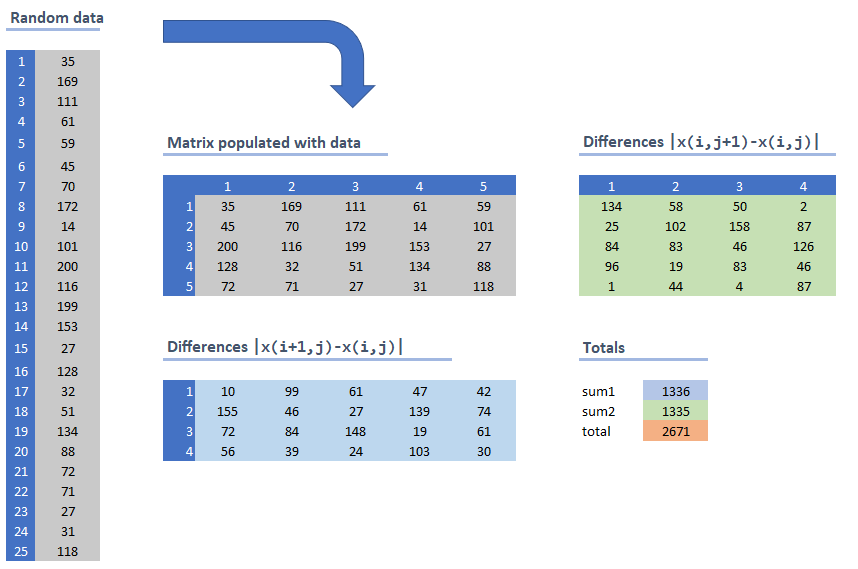Calculation of the objective

The grey matrix is just taking our original random data and lays them out row wise. The light blue matrix elements are the differences $$|x_{i+1,j}-x_{i,j}|$$ while the light green area contains the differences: $$|x_{i,j+1}-x_{i,j}|$$. The objective sums all these differences.

The idea is to find a different ordering of the data into the matrix such that the total sum of differences is minimized.

Trying out all permutations is not really feasible: $25! =15511210043330985984000000$

#### A first conceptual model

To distribute our numbers $$p_k$$ to a matrix $$x_{i,j}$$ we can use the concept of an assignment model:\begin{align}& \sum_{i,j} y_{k,i,j} = 1 &&\forall k \\ & \sum_k y_{k,i,j} = 1&&\forall i,j \\ & y_{k,i,j} \in \{0,1\} \end{align} The values can be recovered with $x_{i,j} = \sum_k p_k y_{k,i,j}$ We used here the assignment model paradigm to implement a permutation of a data vector and place them in a matrix layout. The complete model can look like:

High-level model
\begin{align} \min & \sum_{i\lt n,j} |\color{DarkRed} x_{i+1,j}-\color{DarkRed} x_{i,j}| + \sum_{i,j \lt n} |\color{DarkRed} x_{i,j+1}-\color{DarkRed} x_{i,j}|\\ & \sum_{i,j} \color{DarkRed} y_{k,i,j} = 1 &&\forall k \\ & \sum_k \color{DarkRed} y_{k,i,j} = 1 &&\forall i,j \\ & \color{DarkRed}x_{i,j} = \sum_k \color{DarkBlue}p_k \color{DarkRed} y_{k,i,j} \\ & \color{DarkRed} y_{k,i,j} \in \{0,1\} \end{align}

Note that I protected the terms of the objective against out-of-range indexing.

The original problem allowed the data vector $$p_k$$ to have more elements than the matrix $$x_{i,j}$$. This is easily fixed in our model. Rewrite the assignment constraints as: \begin{align} & \sum_{i,j} y_{k,i,j} \le 1 &&\forall k \\ & \sum_k y_{k,i,j} = 1 &&\forall i,j \end{align} In the following, I will focus on our sample data, which has the length of  $$p_k$$ equal to $$n^2$$.

#### Linearization

This model can be easily linearized, e.g. by:

Linearized model
\begin{align} \min & \sum_{i\lt n,j} \color{DarkRed} d^d_{i,j} + \sum_{i,j \lt n} \color{DarkRed} d^r_{i,j}\\ & \sum_{i,j} \color{DarkRed} y_{k,i,j} = 1 &&\forall k \\ & \sum_k \color{DarkRed} y_{k,i,j} = 1 &&\forall i,j \\ & \color{DarkRed}x_{i,j} = \sum_k \color{DarkBlue}p_k \color{DarkRed} y_{k,i,j} \\ & - \color{DarkRed}d^d_{i,j} \le \color{DarkRed} x_{i+1,j}-\color{DarkRed} x_{i,j} \le \color{DarkRed}d^d_{i,j} &&\forall i\lt n,j \\ & - \color{DarkRed}d^r_{i,j} \le \color{DarkRed} x_{i,j+1}-\color{DarkRed} x_{i,j} \le \color{DarkRed}d^r_{i,j} &&\forall i,j\lt n \\ & \color{DarkRed} y_{k,i,j} \in \{0,1\} \\ & \color{DarkRed} d^d_{i,j}\ge 0,\color{DarkRed} d^r_{i,j}\ge 0 \end{align}

The variables $$d^d_{i,j}$$ indicate the differences downward and the variables $$d^r_{i,j}$$ are the differences when we look to the right.

This turns out to be a terribly difficult model to solve, even for our small data set. With a $$5 \times 5$$ matrix we end up with $$25\times 25= 625$$ binary $$y$$ variables. This is normally a piece of cake for a good MIP solver. But, in fact, I was unable to solve this model to optimality with this data set by any MIP solver I tried. Even after several hours the gap was still substantial. We are in big, big trouble here.

We can add the extra constraint: $\sum_{i,j} x_{i,j} = \sum_k p_k$ This did not seem to make much difference.

#### A good initial solution

A simple heuristic is to sort the data and place the sorted $$p_k$$ accordingly in the matrix. This gives a good initial solution, although it is not optimal.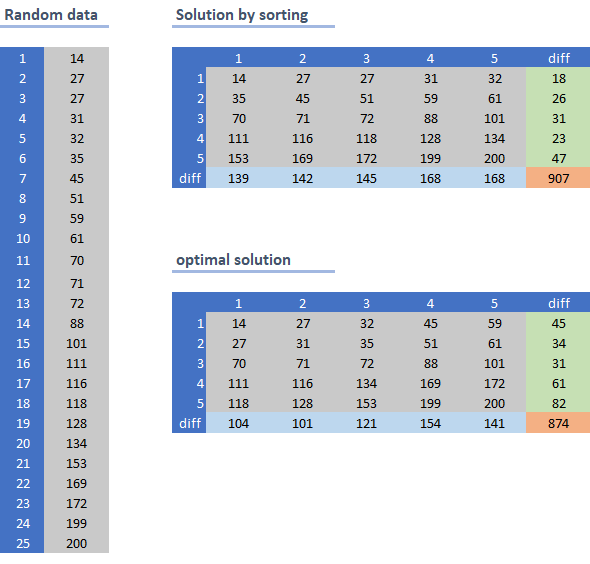Sorting gives a good initial solution

#### Non-decreasing values

In the above picture the values in the matrix were non-decreasing both row-wise and column wise, i.e.  \begin{align} &x_{i,j} \le x_{i+1,j}\\ &x_{i,j} \le x_{i,j+1}\end{align} Intuitively, it makes sense to organize things like that.  For a 1d vector this is somewhat obvious. For a 2d matrix things are a bit more complex, but it makes sense to start with the smallest value in the left upper corner cell $$x_{1,1}$$, and end with the largest number in the right lower cell $$x_{n,n}$$.

If we explicitly add the constraints that values $$x_{i,j}$$ are both row wise and column wise non-decreasing, we achieve a number of things. First we can simplify the calculation of the differences:

Non-decreasing model
\begin{align} \min & \sum_{j} \color{DarkRed} d^d_{j} + \sum_{i} \color{DarkRed} d^r_{i} \\ & \sum_{i,j} \color{DarkRed} y_{k,i,j} = 1 &&\forall k \\ & \sum_k \color{DarkRed} y_{k,i,j} = 1 &&\forall i,j \\ & \color{DarkRed}x_{i,j} = \sum_k \color{DarkBlue}p_k \color{DarkRed} y_{k,i,j} \\ & \color{DarkRed}d^d_{j} = \color{DarkRed} x_{n,j}-\color{DarkRed} x_{1,j} && \forall j\lt n \\ & \color{DarkRed}d^r_{i} = \color{DarkRed} x_{i,n}-\color{DarkRed} x_{i,1} && \forall i\lt n\\ & \color{DarkRed}x_{i,j} \le \color{DarkRed}x_{i+1,j} && \forall i\lt n,j\\ & \color{DarkRed}x_{i,j} \le \color{DarkRed}x_{i,j+1} && \forall i,j\lt n\\ & \color{DarkRed} y_{k,i,j} \in \{0,1\} \\ & \color{DarkRed} d^d_{j}\ge 0,\color{DarkRed} d^r_{i}\ge 0 \end{align}

Of course, with this assumption, we can fix \begin{align} & x_{1,1} = \min_k p_k \\ & x_{n,n} = \max_k p_k \end{align}

This model solves very fast, and we get the solution depicted above (marked by "optimal solution"). I believe this is the optimal solution for our overall problem, but I have not proven this mathematically.

In the comments a different model is suggested:

\begin{align} \min & \sum_{k,k'} \left[ |\color{DarkBlue} p_k - \color{DarkBlue} p_{k'} | \sum_{i,j} \left( \color{DarkRed}y_{k,i,j}\color{DarkRed}y_{k',i+1,j} + \color{DarkRed}y_{k,i,j}\color{DarkRed}y_{k',i,j+1} \right) \right] \\ & \sum_{i,j} \color{DarkRed} y_{k,i,j} = 1 &&\forall k \\ & \sum_k \color{DarkRed} y_{k,i,j} = 1 &&\forall i,j \\ & \color{DarkRed} y_{k,i,j} \in \{0,1\} \end{align}

Assume zeros when indexing out-of-range, i.e. $$y_{k,n+1,j}=y_{k,i,n+1}=0$$. This model can be linearized manually (or this can be left to the solver: e.g. Cplex will linearized this automatically). Still not able to find a proven optimal solution in a short time.

#### A Constraint Programming formulation

Would a CP model help? First, the formulation can be very different than our MIP model:

• We can use high-level global constraints such as all-different and element (use a variable as an index)
• Everything is integer valued in our problem, which makes CP more viable,
Here is an attempt in Minizinc:

 include "globals.mzn"; int: n=5; int: n2=n*n; array[1..n,1..n] of var int : indx; array[1..n,1..n] of var int : x; var int: z; array[1..n2] of int: p; p = [35,169,111,61,59,45,70,172,14,101,200,116,199,153,27, 128,32,51,134,88,72,71,27,31,118]; constraint forall (i,j in 1..n) (indx[i,j]>=1); constraint forall (i,j in 1..n) (indx[i,j]<=n2); constraint alldifferent([indx[i,j]|i,j in 1..n]); constraint forall (i,j in 1..n) (x[i,j] = p[indx[i,j]]); constraint z =    sum(i in 1..n-1,j in 1..n) (abs(x[i+1,j]-x[i,j])) +    sum(i in 1..n,j in 1..n-1) (abs(x[i,j+1]-x[i,j])); solve minimize z;

The indx[i,j] array is a permutation of $$1,\dots,n^2$$. This is easily implemented using the all-different constraint. This index array can be used to find the values $$x_{i,j}$$ using the so-called element constraint: x[i,j] = p[indx[i,j]]. We use here a decision variable indx as an index of a data vector p.

Secondly, we need to solve this. Although the formulation was easy, solving this (small) problem is not trivial. The standard Minizinc solvers did not get close to our "optimal" solution.

#### Conclusion

The problem of populating a matrix with 25 numbers such that the difference between adjacent cells is minimized, turns out to be maddeningly difficult. Adding ordering conditions: values are non-decreasing row- and column-wise makes the model easy to solve. I don't believe we cut off the optimal solution with this, but I have no formal proof of this.

#### References

1. Algorithm to organize a matrix so that neighbors are closest, https://stackoverflow.com/questions/54216526/algorithm-to-organize-a-matrix-so-that-neighbors-are-closest

## Tuesday, January 15, 2019

### Preferences for houses and neighborsIn  the following problem was posted:

• We have $$n$$ houses (denoted by index $$j$$)
• and $$m$$  tenants (using index $$i$$).
• Each tenant $$i$$ has a certain preference for a given house. The tenant can express this by providing three preferred houses: 1 for most preferred, and 2 for second most preferred and 3 for the third preferred house.
• Each tenant can also provide three preferred neighbors, using the same scheme: 1 for most preferred neighbor and 2 or 3 for less preferred.

Let's try to build a model for this.

#### Data

The first thing is I assume $$n\ge m$$: the number of houses exceeds the number of tenants.

To make the objective work, we need to decide what score to use when a house or neighbor is not preferred. We cannot just use zero for this, as 1 is most preferred. We can use the number 4, i.e.: 1 is most preferred, through 4: not preferred and then minimize the achieved score.

I like to use 0 as the default however. For this, I recode the preferences as 3 being the most preferred, and 0 being not preferred. Using zero as the default will lead to sparser models.

I generated some random data with 8 tenants and 10 houses:

----     40 PARAMETER hpref  preference for house, recoded, 3=first,2=second,1=third

house1      house2      house3      house4      house6      house7      house8      house9     house10

tenant1                       1                                   3                                   2
tenant2           1                                               2                                               3
tenant3                       1           3                                   2
tenant4                       1                                   2                                   3
tenant5                       2                       1           3
tenant6                                   1                       2                       3
tenant7           1           3                       2
tenant8                                   3                                   1           2

----     40 PARAMETER npref  preference for neighbor, recoded, 3=first,2=second,1=third

tenant1     tenant2     tenant3     tenant4     tenant5     tenant6     tenant7     tenant8

tenant1                       2           1                                               3
tenant2                                                           1                       3           2
tenant3                       3                       2                       1
tenant4                       1                                               2           3
tenant5                       1           3                                               2
tenant6           2                       3           1
tenant7                                   3                       2           1
tenant8           1                                               3           2


The parameter npref can be interpreted as: $\mathit{npref}_{i,i'} = \text{preference provided by tenant i for having tenant i' as neighbor}$ So each row should have three nonzero entries. This matrix is not symmetric. The benefit of having tenants $$i$$ and $$i'$$ as neighbors is $\mathit{npref}_{i,i'}+\mathit{npref}_{i',i}$ The matrix composed of values $$\mathit{npref}_{i,i'}+\mathit{npref}_{i',i}$$ is symmetric. I assume $$\mathit{npref}_{i,i}=0$$, i.e. the diagonal is zero.

In the following I will consider the preferences as a cardinal utility: we can add things up. Theoretically, the preferences may be more like an ordinal utility: only comparison is defined. I will sidestep this issue to make things easier for me.

#### Assignment

The main variables of the model are: $x_{i,j} = \begin{cases} 1 & \text{if tenant i is assigned to house j} \\ 0 & \text{otherwise}\end{cases}$ The assignment constraints can look like: \begin{align} & \sum_j x_{i,j} = 1 & \forall i \\ & \sum_i x_{i,j} \le 1 & \forall j \end{align} This will be the backbone of the model.

#### Housing preferences

The objective related to housing preferences is simple: $\max \> \sum_{i.j} \mathit{hpref}_{i,j} x_{i,j}$

#### Neighbor preferences

The objective that deals with preferences for neighbors, is more complicated. Let's assume all houses are in one long row. Then we can write: $\max \> \sum_{i,i',j} (\mathit{npref}_{i,i'}+\mathit{npref}_{i',i}) x_{i,j} x_{i',j+1}$ This is a quadratic objective. As we shall see later, it can be easily linearized.

#### Complete model

The complete model can look like:

\begin{align} \max \> & \color{DarkBlue} \lambda \color{DarkRed} f_1 + (1-\color{DarkBlue}\lambda) \color{DarkRed} f_2 \\ & \color{DarkRed} f_1 = \sum_{i.j} \color{DarkBlue}{ \mathit{hpref}}_{i,j} \color{DarkRed} x_{i,j} \\ & \color{DarkRed} f_2 = \sum_{i,i',j} \color{DarkBlue}{(\mathit{npref}}_{i,i'}+\color{DarkBlue}{\mathit{npref}}_{i',i}) \color{DarkRed}x_{i,j} \color{DarkRed}x_{i',j+1} \\ & \sum_j \color{DarkRed} x_{i,j} = 1 & \forall i \\ & \sum_i \color{DarkRed} x_{i,j} \le 1 & \forall j \\ & \color{DarkRed} x_{i,j} \in \{0,1\} \end{align}

Here $$\lambda\gt 0$$ is a weight. With $$\lambda = 0.8$$ (most weight on the housing preferences) I see as solution:

----     79 VARIABLE x.L  assignment

house2      house3      house4      house6      house7      house8      house9     house10

tenant1                                           1.000
tenant2                                                                                           1.000
tenant3                                                       1.000
tenant4                                                                               1.000
tenant5       1.000
tenant6                                                                   1.000
tenant7                               1.000
tenant8                   1.000

----     88 PARAMETER hpoints  preference points for house allocation

house2      house3      house4      house6      house7      house8      house9     house10

tenant1                                           3.000
tenant2                                                                                           3.000
tenant3                                                       2.000
tenant4                                                                               3.000
tenant5       2.000
tenant6                                                                   3.000
tenant7                               2.000
tenant8                   3.000

----     88 PARAMETER npoints  preference points for neighbors

tenant2     tenant3     tenant4     tenant6     tenant8

tenant1                   1.000
tenant3                                           4.000
tenant4       1.000
tenant5                                                       3.000
tenant6                               3.000


We can see that tenant3 and tenant6 are neigbors (house 7 and 8) and thus get 4 bonus points.

A solver like Cplex will be able to solve this model as given. There are some reformulations performed under the hood, as the problem as stated is actually non-convex.

Note: some other solvers will refuse this model as a quadratic equality constraint is non-convex. We can fix this by substituting out $$f_2$$. Other solvers will still refuse to solve the model after this reformulation: the Q matrix is not positive definite, while some other solvers recognize this can be linearized. Convex QP/MIQP solvers are not in agreement about what constitutes an acceptable model.

If the houses are not in a single row, the definition of a neighbor may be a bit more complicated. We can define the parameter $\vartheta_{j,j'} = \begin{cases} 1 & \text{if houses j and j' (with j'\gt j) are neighbors}\\ 0 & \text{otherwise}\end{cases}$ and use as objective: $\max \> \sum_{i,i',j,j'} (\mathit{npref}_{i,i'}+\mathit{npref}_{i',i}) \vartheta_{j,j'} x_{i,j} x_{i',j'}$ It is noted that we need to watch out for double counting. Hence $$\vartheta_{j,j'}= 0$$ for $$j'\le j$$.

#### Linearization

If you only have access to a MIP solver, this problem can be easily reformulated into a linear MIP model by observing that $$y_{i,i',j} = x_{i,j} x_{i',j+1}$$ can be linearized as: \begin{align} & y_{i,i',j} \le x_{i,j} \\ & y_{i,i',j} \le x_{i',j+1} \\ & y_{i,i',j} \ge x_{i,j} + x_{i',j+1} - 1 \\& y_{i,i',j} \in \{0,1\} \end{align} Plugging this into our model yields a linear model.

#### References

1. Optimization solution / algorithm for occupying tenants in houses (combinations), https://stackoverflow.com/questions/54146767/optimization-solution-algorithm-for-occupying-tenants-in-houses-combinations

## Sunday, January 6, 2019

### (Pre)solving Killer Sudokus

Killer Sudoku  is a variant of Sudoku where an additional constraint is that cells belonging to a cage (the colored regions in the picture) add up to a given value.Killer Sudoku (from )
As in standard Sudokus, we need to fill each cell with a number $$1,\dots,9$$, and values in each row, column and $$(3 \times 3)$$ box must be unique. In addition, all values in a cage should be unique. In this puzzle we don't see that some cells already have given values: we need to fill-in all the cells. The solution for the above puzzle is:Solution (from )
Using the standard decision variables for Sudoku puzzles: $x_{i,j,k} = \begin{cases} 1 & \text{if cell (i,j) has value k} \\ 0 & \text{otherwise}\end{cases}$ we can solve this easily with a MIP model. In the model below we use two data structures:

• a mapping $$\mathit{amap}(a,i,j)$$ between area $$a$$ (an area is a row, column or box) and cells $$(i,j)$$: $$\mathit{amap}(a,i,j)$$ is $$\mathit{true}$$ if cell $$(i,j)$$ belongs to area $$a$$,
• a similar mapping $$\mathit{cmap}(c,i,j)$$ between a cage $$c$$ and cell numbers $$(i,j)$$.
With this we can form a compact model:

Killer Sudoku model
\begin{align} & \sum_k \color{DarkRed} x_{i,j,k} = 1 && \forall i,j && \text{One value per cell}\\ & \sum_{i,j| \color{DarkBlue}{\mathit{amap}}(a,i,j)} \color{DarkRed} x_{i,j,k} = 1 && \forall a,k && \text{Unique values in each row, column and box} \\ & \sum_{i,j| \color{DarkBlue}{\mathit{cmap}}(c,i,j)} \color{DarkRed} x_{i,j,k} \le 1 && \forall c,k && \text{Unique values in each cage}\\ & \sum_{i,j,k| \color{DarkBlue}{\mathit{cmap}}(c,i,j)} \color{DarkBlue} k \cdot \color{DarkRed} x_{i,j,k} = \color{DarkBlue} {\mathit{CageSum}}_c && \forall c && \text{Cell values add up to cage sum} \\ & \color{DarkRed} x_{i,j,k} \in \{0,1\} \end{align}

There is no objective in this model: we are just looking for a feasible integer solution.

#### Implementation

The equivalent GAMS model can look like:

 set   a     'area' /i1*i9,j1*j9,b1*b9/   i(a)  'rows' /i1*i9/   j(a)  'columns' /j1*j9/   b(a)  'boxes' /b1*b9/   amap(a,i,j) 'mapping area<->cells'   k     'values' /1*9/ ; * * populate amap * rows, columns, and boxes a.k.a nonets * amap(i,i,j) = yes; amap(j,i,j) = yes; amap(b,i,j) = ord(b) = 3*ceil(ord(i)/3) + ceil(ord(j)/3) - 3; display amap; * * cages * sets   c 'cages' /cage1*cage29/   cmap(c,i,j)  'mapping cage<->cells' ; table cageno(i,j) 'cage numbering'       j1 j2 j3 j4 j5 j6 j7 j8 j9   i1   1  1  2  2  2  3  4  5  6   i2   7  7  8  8  3  3  4  5  6   i3   7  7  9  9  3 10 11 11  6   i4  12 13 13  9 14 10 11 15  6   i5  12 16 16 17 14 10 15 15 18   i6  19 16 20 17 14 21 22 22 18   i7  19 20 20 17 23 21 21 24 24   i8  19 25 26 23 23 27 27 24 24   i9  19 25 26 23 28 28 28 29 29 ; cmap(c,i,j) = ord(c)=cageno(i,j); parameter cagesum(c) /   cage1   3,  cage11  20,  cage21  20   cage2  15,  cage12   6,  cage22   6   cage3  22,  cage13  14,  cage23  10   cage4   4,  cage14  17,  cage24  14   cage5  16,  cage15  17,  cage25   8   cage6  15,  cage16  13,  cage26  16   cage7  25,  cage17  20,  cage27  15   cage8  17,  cage18  12,  cage28  13   cage9   9,  cage19  27,  cage29  17   cage10  8,  cage20   6 /; binary variable x(i,j,k); variable z 'obj'; equation    cellvalue(i,j) 'one value per cell'    unique(a,k)    'unique values in each area'    cunique(c,k)   'unique values in each cage'    cagesums(c)    'summation of cage cells'    obj            'dummy objective' ; cellvalue(i,j).. sum(k, x(i,j,k)) =e= 1; unique(a,k)..    sum(amap(a,i,j),x(i,j,k)) =e= 1; cunique(c,k)..   sum(cmap(c,i,j),x(i,j,k)) =l= 1; cagesums(c)..    sum((cmap(c,i,j),k), k.val*x(i,j,k)) =e= cagesum(c); obj..            z =e= 0; model killer /all/; solve killer using mip minimizing z; parameter v(i,j) 'optimal values'; v(i,j) = sum(k, k.val*x.l(i,j,k)); option v:0; display v;

The function $f(i,j) = 3\left\lceil i/3 \right\rceil + \left\lceil j/3 \right\rceil – 3$ maps $$(i,j)$$ to a box number. We can check this by the fragment:

 set   i /i1*i9/   j /j1*j9/ ; parameter f(i,j) 'box number'; f(i,j) = 3*ceil(ord(i)/3) + ceil(ord(j)/3) - 3; display f;

The output of this fragment is:

----      7 PARAMETER f  box number

j1          j2          j3          j4          j5          j6          j7          j8          j9

i1       1.000       1.000       1.000       2.000       2.000       2.000       3.000       3.000       3.000
i2       1.000       1.000       1.000       2.000       2.000       2.000       3.000       3.000       3.000
i3       1.000       1.000       1.000       2.000       2.000       2.000       3.000       3.000       3.000
i4       4.000       4.000       4.000       5.000       5.000       5.000       6.000       6.000       6.000
i5       4.000       4.000       4.000       5.000       5.000       5.000       6.000       6.000       6.000
i6       4.000       4.000       4.000       5.000       5.000       5.000       6.000       6.000       6.000
i7       7.000       7.000       7.000       8.000       8.000       8.000       9.000       9.000       9.000
i8       7.000       7.000       7.000       8.000       8.000       8.000       9.000       9.000       9.000
i9       7.000       7.000       7.000       8.000       8.000       8.000       9.000       9.000       9.000


The results of the complete MIP model are:

----     75 PARAMETER v  optimal values

j1          j2          j3          j4          j5          j6          j7          j8          j9

i1           2           1           5           6           4           7           3           9           8
i2           3           6           8           9           5           2           1           7           4
i3           7           9           4           3           8           1           6           5           2
i4           5           8           6           2           7           4           9           3           1
i5           1           4           2           5           9           3           8           6           7
i6           9           7           3           8           1           6           4           2           5
i7           8           2           1           7           3           9           5           4           6
i8           6           5           9           4           2           8           7           1           3
i9           4           3           7           1           6           5           2           8           9


Sudokus are typically solved in the presolver: the MIP solver does not need to do any Simplex iterations or Branch-and-Bound nodes. We see the same thing here. The Cplex solver log shows:

 MIP Presolve eliminated 608 rows and 724 columns. MIP Presolve modified 882 coefficients. Aggregator did 6 substitutions. All rows and columns eliminated. Presolve time = 0.11 sec. (5.58 ticks) Proven optimal solution. MIP Solution:            0.000000    (0 iterations, 0 nodes)

CBC is also able to solve this without much sweat:

 Calling CBC main solution routine... No integer variables out of 560 objects (560 integer) have costs branch on satisfied N create fake objective Y random cost Y Integer solution of 0 found by feasibility pump after 0 iterations and 0 nodes (0.94 seconds) Search completed - best objective 0, took 0 iterations and 0 nodes (0.96 seconds)

It looks like CBC is not able to presolve the whole model away. It subsequently invokes the feasibility pump (a heuristic to find feasible solutions quickly).

#### Multiple solutions?

We can easily prove this solution is unique, by adding a cut that forbids this solution, and resolving. I added to the model:

equation cut;
cut..
sum((i,j,k),(2*x.l(i,j,k)-1)*x(i,j,k)) =l= sum((i,j,k),x.l(i,j,k))-1;

Indeed this solution is unique: adding the cut made the model infeasible.

#### Unique values in a cage

Firstly, it is noted that for this data set we could have removed the condition that within a cage we only want unique values. This constraint is non-binding: if we remove it we find the same solution. Even stronger: after removing the cunique constraint, we still get a single, unique solution.

An alternative formulation is to combine the constraints unique and cunique into one big equation For this we add the cages $$c$$ to our amap data structure:

 * * populate amap * rows, columns, boxes and cages * amap(i,i,j) = yes; amap(j,i,j) = yes; amap(b,i,j) = ord(b) = 3*ceil(ord(i)/3) + ceil(ord(j)/3) - 3; amap(c,i,j) = cmap(c,i,j); display amap;

Now the simplified model can look like:

Killer Sudoku model 2
\begin{align} & \sum_k \color{DarkRed} x_{i,j,k} = 1 && \forall i,j && \text{One value per cell}\\ & \sum_{i,j| \color{DarkBlue}{\mathit{amap}}(a,i,j)} \color{DarkRed} x_{i,j,k} \le 1 && \forall a,k && \text{Unique values in each row, column, box and cage} \\ & \sum_{i,j,k| \color{DarkBlue}{\mathit{cmap}}(c,i,j)} \color{DarkBlue} k \cdot \color{DarkRed} x_{i,j,k} = \color{DarkBlue} {\mathit{CageSum}}_c && \forall c && \text{Cell values add up to cage sum} \\ & \color{DarkRed} x_{i,j,k} \in \{0,1\} \end{align}

It requires a bit of thought that we can use a $$\le$$ constraint for all uniqueness constraints.

When we solve this model with Cplex, it is interesting to see that the presolver is not as effective:

 MIP Presolve eliminated 328 rows and 382 columns. MIP Presolve modified 825 coefficients. Aggregator did 7 substitutions. Reduced MIP has 280 rows, 341 columns, and 1645 nonzeros. Reduced MIP has 341 binaries, 0 generals, 0 SOSs, and 0 indicators. Presolve time = 0.05 sec. (7.14 ticks) Found incumbent of value 0.000000 after 0.14 sec. (17.27 ticks)

The message  "All rows and columns eliminated" is notably missing.